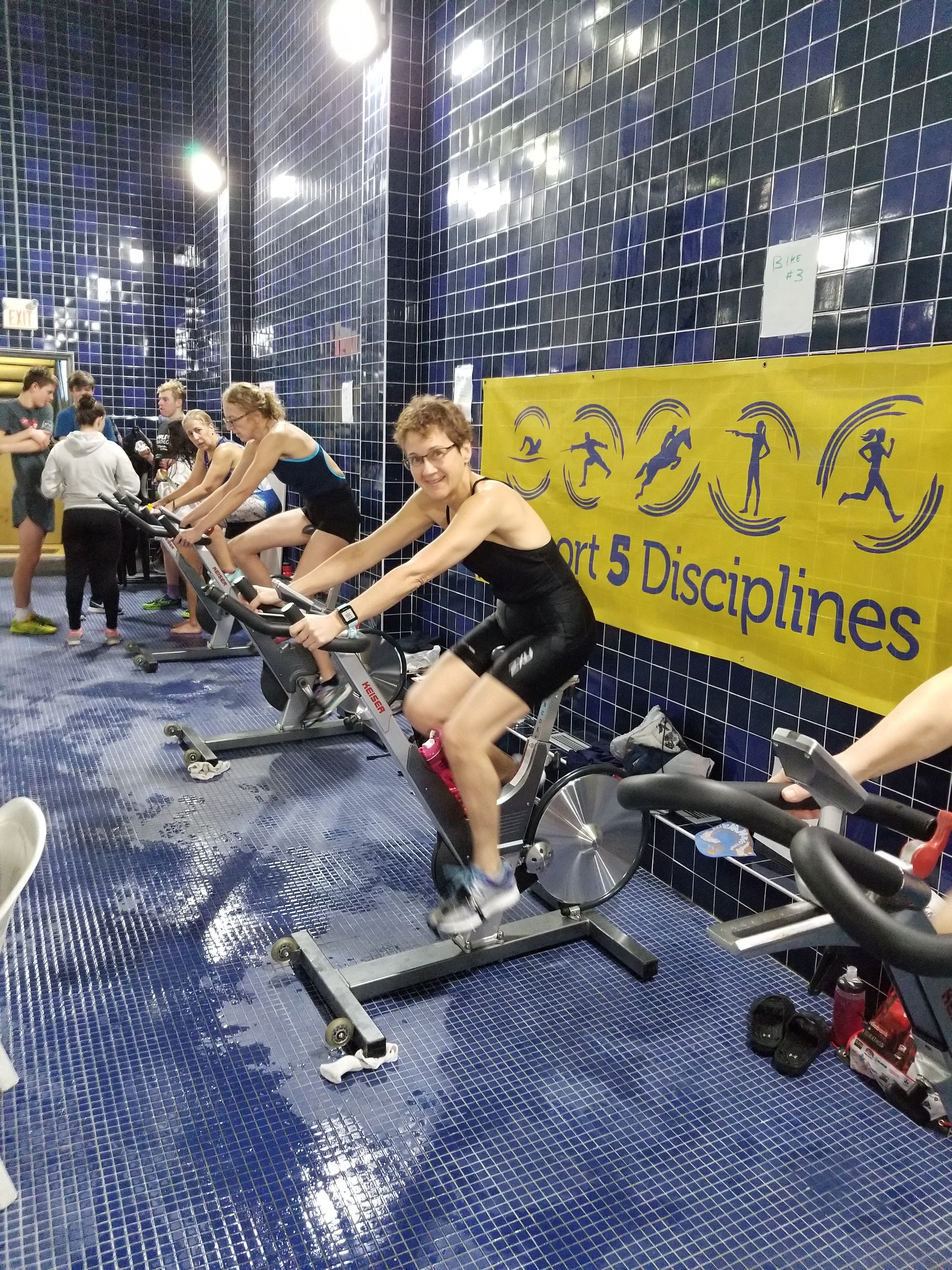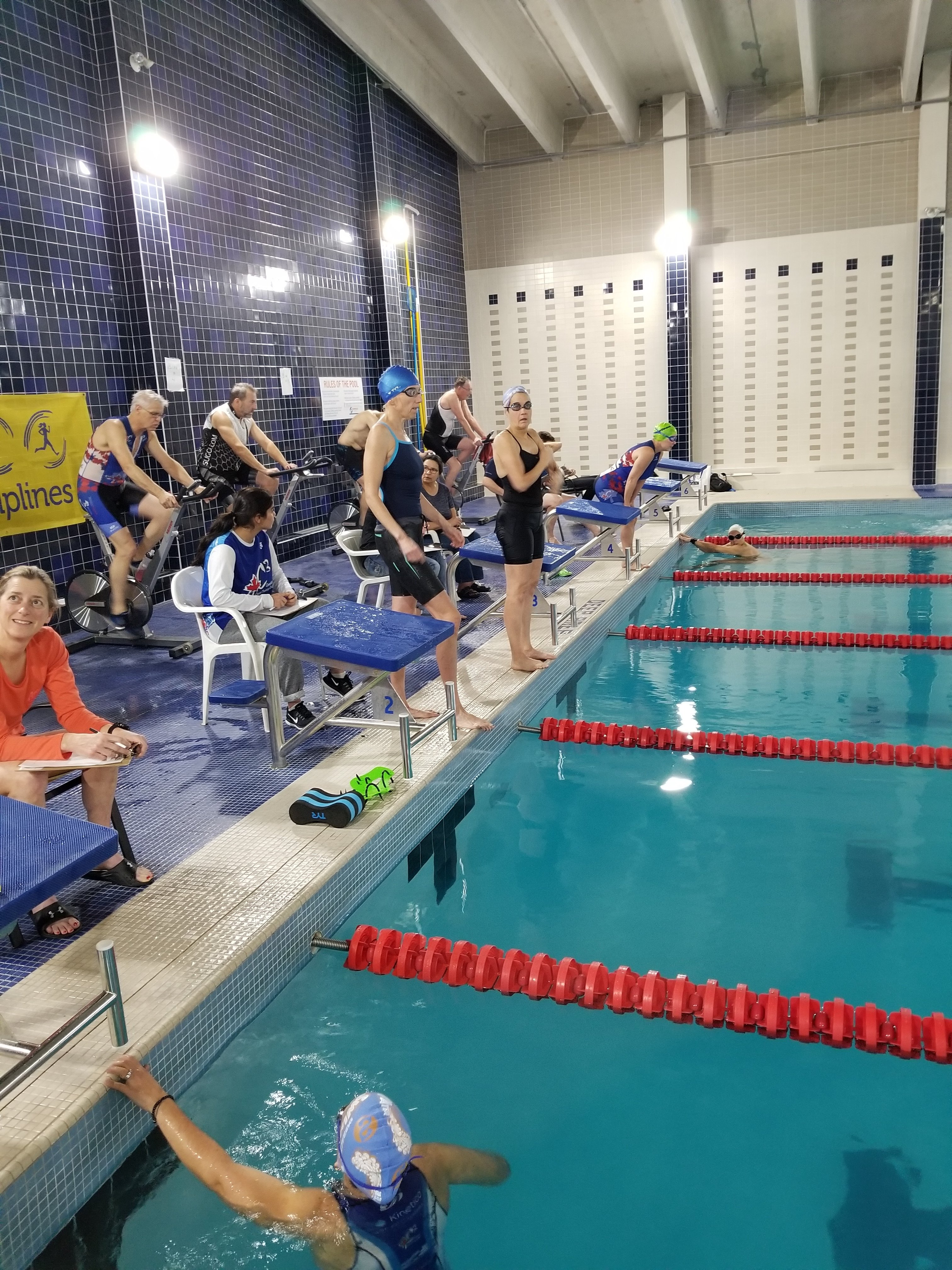•FITNESS CENTRE DESIGN
••PERSONAL COACHING
•VIRTUAL WORKOUTS

PB Indoor Tri 2019 Results - Race #1## Overall Results

Tristen Jones
Junior man
1200 m swim = 900 pts
10.5km bike = 875 pts
4.7km run = 925 pts
Total = 2700 pts

Garrett Mason
Junior man
1075m = 775 pts swim
9.5km bike = 825 pts
4.5km run = 900 pts
Total = 2500 pts

Kristen Marchant
female 25
1150 m swim = 850 pts
10km bike = 850 pts
3.2km run = 750 pts
Total = 2450

Chilyn Fenton
Junior female
1200m swm = 900 pts
9.6km bike = 825 pts
3 km run = 725 pts
Total 2450

Kira Gupta Baltazar
female junior
1200m swim = 900 pts
9.6km bike = 825pts
estimated 2.5km = 650 pts
Total 2375 pts * final run distance estimated

Nissim Abergel
male 47
1000m swim = 700 pts
9.5km bike = 825 pts
3.7km run = 800 pts
Total = 2325 pts

Luis Lopez
male 42
950 m swim = 650 pt
10.3km bike = 850 pts
3.18k run = 725 pts
Total = 2225 pts

Tynan Webb
15 Male (Junior)
Swim 975m = 675 pts
8+ km bike =750 pts
3.6+ km = 800 pts
Total = 2225 pts

Male 53
850m swim = 550 pts
10km = 850 pts
3.6km + = 800 pts
Total = 2200 pts

Michael Latifi
male 25
975 m swim = 675 pts
8.1km swim = 750 pts
4.2km = 875 pts
Total = 2200 pts

Romuald Le Calonnec
Male 33
850 m swim = 550 pts
7.6km bike = 725 pts
4.3km run = 875 pts
Total = 2150 pts

David Uglow
male 61
850 m swim = 550 pts
9+ km = 800 pts
3.4km = 775 pt
Total = 2125 pts

Isac Hall
Male 31
800 swim = 500 pts
8.5km bike = 775 pts
3.6km run = 800 pts
Total = 2075 pts

Caroline Holicka
Female 17
850m swim = 550 pts
8.5km bike = 775 pts
2.4km run = 650 pts
Total pts = 1975 pts

Irina Metelyuk
Female 26
775m swim = 475pts
8km bike = 750 pts
3.3km run = 750 pts
1975 pts

Bob Knuckey
male 70+
750m swim = 450 pts
8km bike = 750 pts
3.2km run = 750 pts
Total = 1950 pts

Geoff Gow
male 47
650m swim = 350pts
9.6km bike = 825pts
3.4km run = 775 pts
Total = 1950 pts

Mauricio Rojas
46 Male
700m swim = 400 pts
9.5km bike = 825 pts
3.0 km run = 725 pts
Total =1950 pts

Arquelle Staveley
female 16
900m swim = 600 pts
7.9km bike = 725pts
2.4km run = 650 pts
Total = 1925 pts

Breandon Peterson
32 male
700m swim = 400 pts
8.2km bike = 750 pts
3.38 run = 750 pts
Total = 1900 pts

Terri O’Sullivan
female 49
700m swim = 400 pts
7.4km bike = 700 pts
3.28km run = 750 pts
Total = 1850 pts

Glen Hall
Male 45
675m swim = 375 pts
8.4km bike = 750 pts
3.13 km run = 725pts
Total = 1850 pts

Caroline Bolduc
Female 46
750m swim = 450 pts
8km bike = 750 pts
2km run = 600 pts
Total = 1800 pts

John (Alex) Hall
male 31
800m swim = 500 pts
7km bike = 700 pts
3.5km run = 775 pts
Total = 1775 pts

Hilary Corbeil
Female 47
700m swim = 400 pts
7.4 km bike = 700 pts
2.6km run = 675 pts
Total = 1775

Jim Reid
male 62
650m swim = 350 pts
7.7km bike = 725 pts
2.84km run = 700 pts
Total = 1775 pts

Lucy Gilmore
Female 56
600m swim = 300 pts
7.8km bike = 725 pts
3km run = 725 pts
Total = 1750 ptts

Doris Neculoiu
Female 51
600m swim = 300 pts
Bike 8.2 km = 750 pts
2.65km run = 675 pts
Total = 1725 pts

Patricia Lopez
Female 50
525m swim = 200 pts
8.6km bike = 775 pts
3+ km run = 725 pts
Total = 1700 pts

Peggy Reid
Female 60
575m swim = 250 pts
8.6km bike = 775 pts
2.4km run = 650 pts
Total = 1675 pts

Julian Kenney
male 64
675m swim = 375 pts
6.8km bike = 700 pts
2km run = 600 pts
Total = 1675 pts

male 44
375m swim = 150 pts
9.1km bike = 800 pts
2.5km run = 650 pts
Total = 1600 pts

Jane Holicka
Female 50
550m swim = 225 pts
7.5+ km bike = 725 pts
2.2km run = 625 pts
Total = 1575 pts

Larry Aspinall
male 72
650m swim = 350 pts
7.1km bike = 700pts
1.3km run = 500 pts
Total = 1550 pts

Tracy Thornycroft
Female 50
625 m swim = 325pts
6.3km bike = 650 pts
1.82km run = 575 pts
Total = 1550 pts

George Holicka
Male 49
650m swim = 350 pts
7+ km bike = 700 pts
2.06 km run = 600 pts
Total = 1550 pts

Karen Robson
Female 46
450 m swim = 125 pts
7.5km bike = 725 pts
2.03km run = 600 pts
Total = 1450 pts

Ann Kim
Female 46
300m swim = 100 pts
8.4km bike = 750 pts
1.71 km run = 550 pts
Total pts = 1400 pts

­